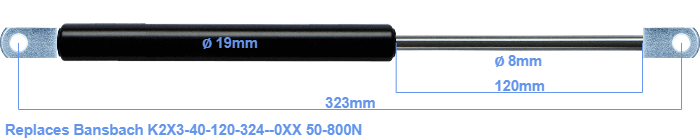# Replacement for Bansbach K2X3-40-120-324–0XX 50-800N

\$31.53

Replacement gas spring for the Bansbach K2X3-40-120-324--0XX 50-800 Newton. The eye has a thickness of 10mm and a hole diameter of 8.1mm. Brand: Stabilus Industry Line.

Please note:
• Mounting part tube: The thickness of the eye of this replacement is 10mm instead of 14mm.
• Mounting part rod : The thickness of the eye of this replacement is 10mm instead of 12mm.
•  Force Choose an option50 Newton60 Newton80 Newton100 Newton120 Newton140 Newton150 Newton160 Newton180 Newton200 Newton220 Newton240 Newton250 Newton260 Newton280 Newton300 Newton320 Newton340 Newton350 Newton360 Newton380 Newton400 Newton420 Newton440 Newton450 Newton460 Newton480 Newton500 Newton520 Newton540 Newton550 Newton560 Newton580 Newton600 Newton620 Newton640 Newton650 Newton660 Newton680 Newton700 Newton720 Newton740 Newton750 Newton760 Newton780 Newton800 NewtonClearThis gas spring has a cylinder diameter of 19 mm. The cross section of the rod is 8 mm. The chrome part is called the rod. It has a length of 120 mm. In total, the length is 323 millimeter. Attention: this is the length between the centers of the mounting parts. Without the mounting parts this gas spring is 285 mm long (thread to thread). This replacement gas spring has a force of 50-800 Newton. This is not an official Bansbach gas spring, but a so called replacement. This gas spring was produced by Stabilus subsidiary Hahn with the brand Stabilus Industry Line. The force (50-800 N) and dimensions are almost entirely identical to the original.